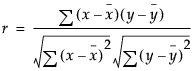Publication date: 04/12/2021

## Pearson Product-Moment Correlation

The Pearson product-moment correlation coefficient measures the strength of the linear relationship between two variables. For response variables X and Y, it is denoted as r and computed as follows:If there is an exact linear relationship between two variables, the correlation is 1 or –1, depending on whether the variables are positively or negatively related. If there is no linear relationship, the correlation tends toward zero.

Want more information? Have questions? Get answers in the JMP User Community (community.jmp.com).